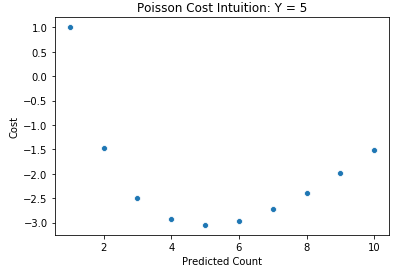# What is the cost function used in Poisson Regression?

Poisson regression uses the following cost function:

wheredenotes the predicted value for the ith observation. The intuition for the cost function can be visualized below, where in this example, the actual count of the observation is 5. As the predicted value moves away from 5, the cost assigned to it increases, corresponding to a poorer prediction.#### What is Beta regression?

Find out all the ways
that you can